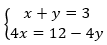# Week 13 Day 1 Opener - A.2.17.1 A Curious System

Andre is trying to solve this system of equations:Looking at the first equation, he thought, "The solution to the system is a pair of numbers that add up to 3. I wonder which two numbers they are."  Choose any two numbers that add up to 3. Let the first one be the x-value and the second one be the y-value.

The pair of values you chose is a solution to the first equation. Check if it is also a solution to the second equation.

How many solutions does the system have? Graph the system of equations to show that you are right.